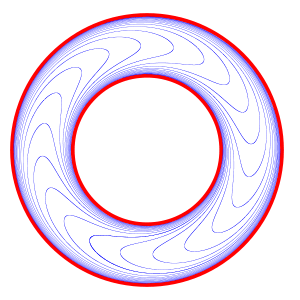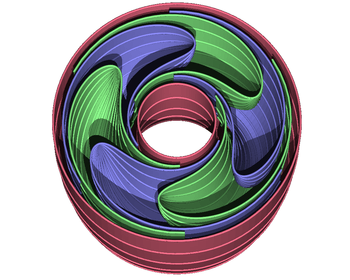# Foliation

For other uses, see Foliation (disambiguation).2-dimensional section of Reeb foliation3-dimensional model of Reeb foliation

In mathematics, a foliation is a geometric tool for understanding manifolds. The leaves of a foliation consist of integrable subbundles of the tangent bundle. Foliating a manifold may split it up into pieces that interact simply. A foliation looks locally like a decomposition of the manifold as a union of parallel submanifolds of smaller dimension.

## Definition

More formally, a dimension p foliation F of an n-dimensional manifold M is a covering by charts Ui together with mapssuch that for overlapping pairs Ui, Uj the transition functions φij : RnRn defined bytake the formwhere x denotes the first np coordinates, and y denotes the last p co-ordinates. That is,In the chart Ui, the stripes x = constant match up with the stripes on other charts Uj. Technically, these stripes are called plaques of the foliation. In each chart, the plaques are p dimensional submanifolds. These submanifolds piece together from chart to chart to form maximal connected injectively immersed submanifolds called the leaves of the foliation.

The notion of leaves allows for a more intuitive way of thinking about a foliation. A p-dimensional foliation of an n-manifold M may be thought of as simply a collection {Ma} of pairwise-disjoint, connected, immersed p-dimensional submanifolds (the leaves of the foliation) of M, such that for every point x in M, there is a chartwith U homeomorphic to Rn containing x such that every leaf, Ma, meets U in either the empty set or a countable collection of subspaces whose images underinare p-dimensional affine subspaces whose first np coordinates are constant.

If we shrink the chart Ui it can be written as Uix × Uiy, where UixRnp, UiyRp, Uiy is homeomorphic to the plaques, and the points of Uix parametrize the plaques in Ui. If we pick y0 in Uiy, then Uix × {y0} is a submanifold of Ui that intersects every plaque exactly once. This is called a local transversal section of the foliation. Note that due to monodromy global transversal sections of the foliation might not exist.

## Examples

### Flat space

Consider an n-dimensional space, foliated as a product by subspaces consisting of points whose first np coordinates are constant. This can be covered with a single chart. The statement is essentially that Rn = Rnp × Rp with the leaves or plaques Rp being enumerated by Rnp. The analogy is seen directly in three dimensions, by taking n = 3 and p = 2: the 2-dimensional leaves of a book are enumerated by a (1-dimensional) page number.

### Bundles

A rather trivial example of foliations are products M = B × F, foliated by the leaves Fb = {b} × F, bB. (Another foliation of M is given by Bf = B × {f } , f F.)

A more general class are flat G-bundles with G = Homeo(F) for a manifold F. Given a representation ρ : π1(B) → Homeo(F), the flat Homeo(F)-bundle with monodromy ρ is given by, where π1(B) acts on the universal coverby deck transformations and on F by means of the representation ρ.

Flat bundles fit into the framework of fiber bundles. A map π : MB between manifolds is a fiber bundle if there is a manifold F such that each bB has an open neighborhood U such that there is a homeomorphismwith, with p1 : U × FU projection to the first factor. The fiber bundle yields a foliation by fibers. Its space of leaves L is homeomorphic to B, in particular L is a Hausdorff manifold.

### Coverings

If MN is a covering map between manifolds, and F is a foliation on N, then it pulls back to a foliation on M. More generally, if the map is merely a branched covering, where the branch locus is transverse to the foliation, then the foliation can be pulled back.

### Submersions

If MnNq, (qn) is a submersion of manifolds, it follows from the inverse function theorem that the connected components of the fibers of the submersion define a codimension q foliation of M. Fiber bundles are an example of this type.

An example of a submersion, which is not a fiber bundle, is given byThis submersion yields a foliation of [−1, 1] × R which is invariant under the Z-actions given byfor (x, y) ∈ [−1, 1] × R and zZ. The induced foliations of Z\([−1, 1] × R) are called the 2-dimensional Reeb foliation (of the annulus) resp. the 2-dimensional nonorientable Reeb foliation (of the Möbius band). Their leaf spaces are not Hausdorff.

### Reeb foliations

Define a submersionwhere (r, θ) ∈ [0, 1] × Sn−1 are cylindrical coordinates on the n-dimensional disk Dn. This submersion yields a foliation of Dn × R which is invariant under the Z-actions given byfor (x, y) ∈ Dn × R, zZ. The induced foliation of Z\(Dn × R) is called the n-dimensional Reeb foliation. Its leaf space is not Hausdorff.

For n = 2, this gives a foliation of the solid torus which can be used to define the Reeb foliation of the 3-sphere by gluing two solid tori along their boundary. Foliations of odd-dimensional spheres S2n+1 are also explicitly known.

### Lie groups

If G is a Lie group, and H is a Lie subgroup, then G is foliated by cosets of H. When H is closed in G, the quotient space G/H is a smooth (Hausdorff) manifold turning G into a fiber bundle with fiber H and base G/H. This fiber bundle is actually principal, with structure group H.

### Lie group actions

Let G be a Lie group acting smoothly on a manifold M. If the action is a locally free action or free action, then the orbits of G define a foliation of M.

### Kronecker foliation

The set of lines on the torus T = R2/Z2 with the same slope θ forms a foliation. The leaves are obtained by projecting straight lines of slope θ in the plane R2 onto the torus. If the slope is rational then all leaves are closed curves homeomorphic to the circle, while if it is irrational, the leaves are noncompact, homeomorphic to the real line, and dense in the torus (cf Irrational rotation). The irrational case is known as the Kronecker foliation, after Leopold Kronecker. A similar construction using a foliation of Rn by parallel lines yields a 1-dimensional foliation of the n-torus Rn/Zn associated with the linear flow on the torus.

### Suspension foliations

A flat bundle has not only its foliation by fibres but also a foliation transverse to the fibers, whose leaves arewhereis the canonical projection. This foliation is called the suspension of the representation ρ : π1(B) → Homeo(F).

In particular, if B = S1 andis a homeomorphism of F, then the suspension foliation ofis defined to be the suspension foliation of the representation ρ : Z → Homeo(F) given by ρ(z) = Φz. Its space of leaves is L = F/~, where x ~ y whenever y = Φn(x) for some nZ.

The Kronecker foliations of the 2-torus are the suspension foliations of the rotations Rα : S1S1 by angle α ∈ [0, 2π).

## Foliations and integrability

There is a close relationship, assuming everything is smooth, with vector fields: given a vector field X on M that is never zero, its integral curves will give a 1-dimensional foliation. (i.e. a codimension n − 1 foliation).

This observation generalises to the Frobenius theorem, saying that the necessary and sufficient conditions for a distribution (i.e. an np dimensional subbundle of the tangent bundle of a manifold) to be tangent to the leaves of a foliation, is that the set of vector fields tangent to the distribution are closed under Lie bracket. One can also phrase this differently, as a question of reduction of the structure group of the tangent bundle from GL(n) to a reducible subgroup.

The conditions in the Frobenius theorem appear as integrability conditions; and the assertion is that if those are fulfilled the reduction can take place because local transition functions with the required block structure exist. For example, in the codimension 1 case, we can define the tangent bundle of the foliation as ker(α), for some (non-canonical) α ∈ Ω1 (i.e. a non-zero co-vector field). A given α is integrable iff α = 0 everywhere.

There is a global foliation theory, because topological constraints exist. For example, in the surface case, an everywhere non-zero vector field can exist on an orientable compact surface only for the torus. This is a consequence of the Poincaré–Hopf index theorem, which shows the Euler characteristic will have to be 0. There are many deep connections with contact topology, which is the "opposite" concept.

## Existence of foliations

Haefliger (1970) gave a necessary and sufficient condition for a distribution on a connected non-compact manifold to be homotopic to an integrable distribution. Thurston (1974, 1976) showed that any compact manifold with a distribution has a foliation of the same dimension.